# Write a balanced overall reaction from these unbalanced half-reactions. Cu rightarrow cu2+ Ag+ rightarrow

Write a balanced overall reaction from these unbalanced half-reactions.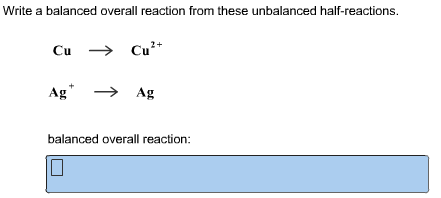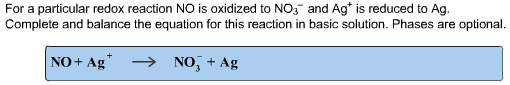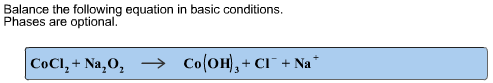Concepts and reason
The basic concept is the balancing of equations. The following steps are used to balance the chemical equation. Write the correct chemical formula of each compound present in the equation. Verify the net ionic charge and balance it. Balance the number of atoms on each side of the equation by adding least coefficient. Balance H and O at the last.

Fundamentals
According to law of conservation of mass “Matter neither be created nor be destroyed”. Therefore the number of each type of atom on each side of a chemical equation must be the same.

Part 1
The half reactions are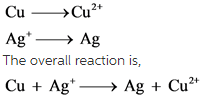The net charge is not balanced. To balance this, add 2 in front of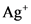in reactant side. Then add 2 in front of Ag in product side to balance silver atom.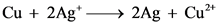Part 2
Since the reaction takes place in basic medium, one can add OH- (if required) anywhere in the chemical equation.
The reaction is,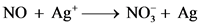It looks like a redox reaction. In this reaction, oxidation state of N changes from +2 to +5. It indicates that it loses three electrons. To receive these threeelectrons, three ions are required. So add coefficient 3 toas well as Ag.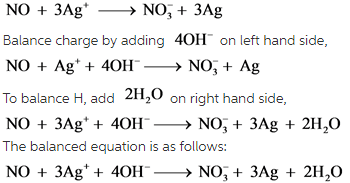In the first step, number of electrons involved are balanced by adding 3 to Ag and. Next net charge is balanced by adding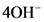. Then number of H is balanced by adding 2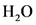.

Part 3
It looks like a redox reaction. In this reaction, oxidation state of Co changes from +2 to +3 and O is from -1 to -2. It indicates that O gains two electrons. To supply these three electrons, two Co are required. So add coefficient 2 to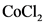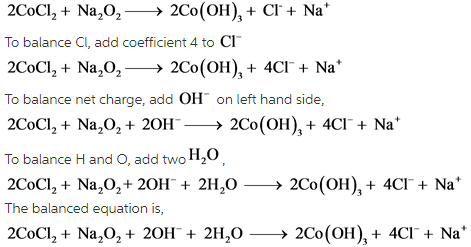.

In the first step, number of electrons involved are balanced by adding 2 to. Next net charge is balanced by adding 2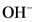. Then number of H and O are balanced by adding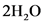.